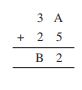# Q2. Find the values of the letters in each of the following and give reasons for the steps involved.Here

answer's unit place = 3 , possible addition of unit places digit = 13

A + 8 = 13

A = 13 - 8 = 5

remainder = 1

Ten's place of answer  = 4 + 9 + 1 =  14

B = 4

remainder = 1

100's place = C = 1

Hence value of  A = 5 , B = 4 and C = 1.

Exams
Articles
Questions